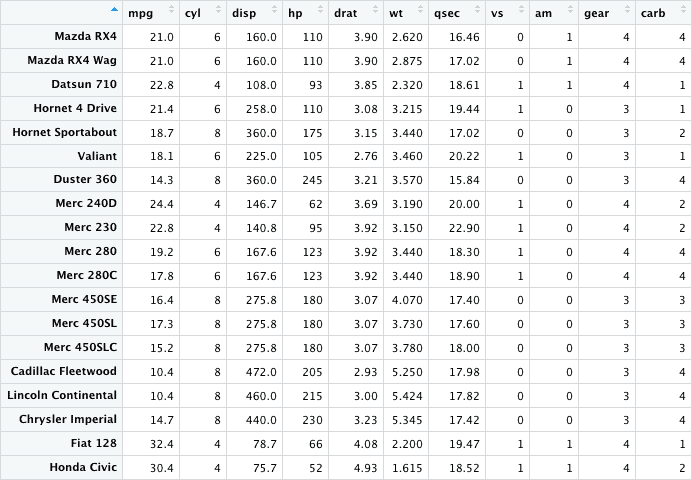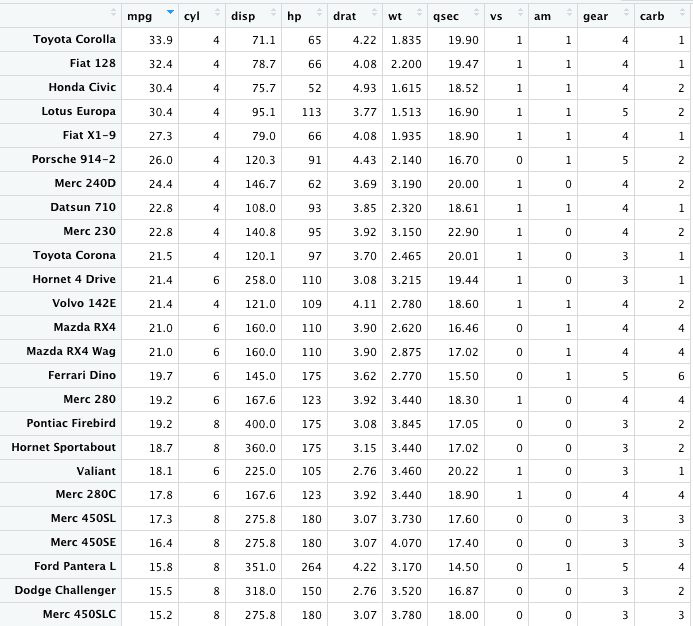# View in R: How to Use View() Function in R

R language lets you save data by storing it inside an R object. But, What’s an object? Well, An object is just a name that you can use to call up stored data. Wherever R encounters the object, it will replace it with the data saved inside.

## View in R

The View() function in R invokes a spreadsheet-style data viewer on a matrix-like R object. To view all the contents of a defined object, use the View() function. Behind the scenes, the R calls utils::View() on the input and returns it invisibly.

If the input is not a data frame, it is processed using a variant of as.data.frame(head(x, n)). A message is printed if the number of rows exceeds n. This function does not affect non-interactive sessions.

### Syntax

``View(ObjectName, title)``

### Parameters

ObjectName: It is an R object coerced to a data frame with non-zero numbers of rows and columns.

title: It is a title for a viewer window. Defaults to the ObjectName prefixed by Data.

### Return Value

It returns an Invisible NULL. The functions put up a window and return immediately: the window can be closed via its controls or menus.

### Example

To demonstrate the View() function, you need to have to install RStudio on your machine. Open the RStudio.

Create an R file and add the following code.

``````df <- structure(list(year = c(2016, 2017, 2018, 2019), length_days = c(365.32, 366.41, 366.53, 364.95)),
.Names = c("year", "days"),
class = "data.frame",
row.names = c(NA, -4L))

View(df)``````

In this example, we are creating a data frame using the structure() function.

To see the output, run the above code inside the RStudio, and it will give you the following output.## Viewing the mode of an object

To find what type of data is contained within the object, use the mode() function. The mode of an object can be viewed using the mode function.

``````df <- structure(list(year = c(2016, 2017, 2018, 2019), length_days = c(365.32, 366.41, 367.53, 368.95)),
.Names = c("year", "days"),
class = "data.frame",
row.names = c(NA, -4L))

mode(df)

``````

#### Output

`` "list"``

## Viewing the class of an object

The possible values for “matrix“, “array“, “factor” and “data.frame” objects.  The class of an object can be viewed with the class function.

``````df <- structure(list(year = c(2016, 2017, 2018, 2019), length_days = c(365.32, 366.41, 367.53, 368.95)),
.Names = c("year", "days"),
class = "data.frame",
row.names = c(NA, -4L))

class(df)``````

#### Output

`` "data.frame"``

## Getting Information on a Dataset

There are several functions for listing the contents of an object or dataset.

1. To list the objects in the working environment, use the ls() function.
2. To list the variables in your data, use the names() function.
3. To list the structure of your data, use the str() method.
4. To list the levels of factors in your data, use the levels() function.
5. To get the dimension of the object, use the dim() method.
6. To get the class of the object, use the class() method.
7. To get the first n number of rows and columns of the dataset, use the head() function.
8. To get the last n number of rows and columns of the dataset, use the tail() function.

## View function in R is not working.

If you can’t view the data frame using the View() function, you often think that the view function is not working properly in R., But in reality, there could be a version problem you are using because it is not supported.

If you are using, for example, R Version 3.1.2 with RStudio Version 0.98.1091, then the View() function from the “utils” package is not supported. So to solve this, you should upgrade your RStudio.

So, often you can solve this type of error by updating your packages related to R.

## View function in RStudio

RStudio includes a data viewer that allows you to look inside data frames and other rectangular data structures.

You can invoke the viewer in a console by calling the View() function on the data frame you want to look at. For instance, to view the built-in mtcars dataset, run these commands.

``````data(mtcars)
View(mtcars)``````

#### OutputYou can also sort the data of the viewer. You can sort by any column by just clicking on the column. Then, click on a column that’s already sorted to reverse the sort direction.We have sorted the column mpg in descending order.

You can also perform searching, filtering, saving filters, and auto-refreshing. For more information, please check out the data viewer guide for RStudio.

## Conclusion

In this example, we have seen the View() method and how to use it to see the data objects in R. Then, we have seen the mode and class of View() and finally seen the View() in RStudio.

That is it for the View() function in R.

Categories R